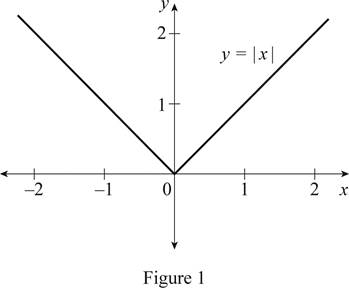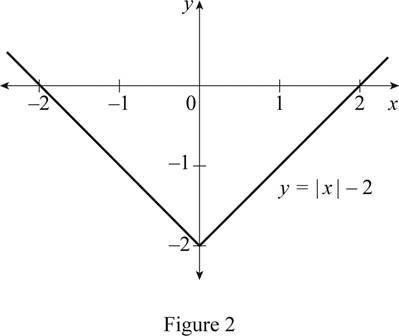# The function y = | x | by hand without plotting any points and then graph of the function y = | x | − 2 .### Single Variable Calculus: Concepts...

4th Edition
James Stewart
Publisher: Cengage Learning
ISBN: 9781337687805### Single Variable Calculus: Concepts...

4th Edition
James Stewart
Publisher: Cengage Learning
ISBN: 9781337687805

#### Solutions

Chapter 1.3, Problem 20E
To determine

## To sketch: The function y=|x| by hand without plotting any points and then graph of the function y=|x|−2 .

Expert Solution

### Explanation of Solution

The standard graph of the function y=|x| is roughly drawn as shown below in Figure 1.Then, draw the graph of y=|x|2 by shifting the graph y=|x| by 2 units downward. Thus, the graph of y=|x|2 is shown below in Figure 2.Observe that Figure 2 is obtained from Figure 1 in such a way that it is shifted downward by 2 units.

### Have a homework question?

Subscribe to bartleby learn! Ask subject matter experts 30 homework questions each month. Plus, you’ll have access to millions of step-by-step textbook answers!